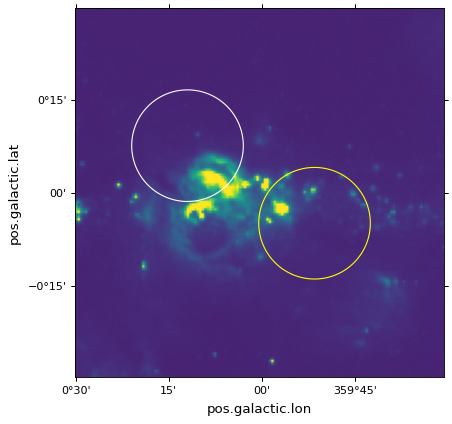# Overplotting markers and artists¶

For the example in the following page we start from the example introduced in Initializing axes with world coordinates.

## Pixel coordinates¶

Apart from the handling of the ticks, tick labels, and grid lines, the `WCSAxes` class behaves like a normal Matplotlib `Axes` instance, and methods such as `imshow()`, `contour()`, `plot()`, `scatter()`, and so on will work and plot the data in pixel coordinates by default.

In the following example, the scatter markers and the rectangle will be plotted in pixel coordinates:

```# The following line makes it so that the zoom level no longer changes,
# otherwise Matplotlib has a tendency to zoom out when adding overlays.
ax.set_autoscale_on(False)

# Add a rectangle with bottom left corner at pixel position (30, 50) with a
# width and height of 60 and 50 pixels respectively.
from matplotlib.patches import Rectangle
r = Rectangle((30., 50.), 60., 50., edgecolor='yellow', facecolor='none')

# Add three markers at (40, 30), (100, 130), and (130, 60). The facecolor is
# a transparent white (0.5 is the alpha value).
ax.scatter([40, 100, 130], [30, 130, 60], s=100, edgecolor='white', facecolor=(1, 1, 1, 0.5))
```

(png, svg, pdf)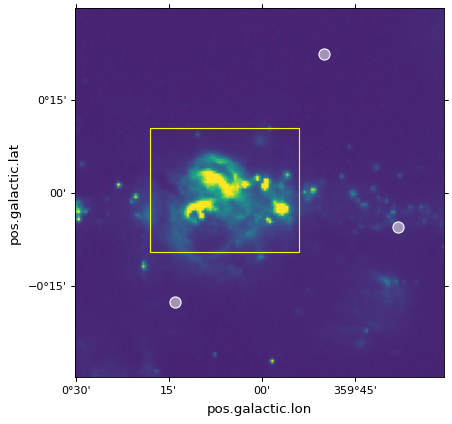## World coordinates¶

All such Matplotlib commands allow a `transform=` argument to be passed, which will transform the input from world to pixel coordinates before it is passed to Matplotlib and plotted. For instance:

```ax.scatter(..., transform=...)
```

will take the values passed to `scatter()` and will transform them using the transformation passed to `transform=`, in order to end up with the final pixel coordinates.

The `WCSAxes` class includes a `get_transform()` method that can be used to get the appropriate transformation object to convert from various world coordinate systems to the final pixel coordinate system required by Matplotlib. The `get_transform()` method can take a number of different inputs, which are described in this and subsequent sections. The two simplest inputs to this method are `'world'` and `'pixel'`.

For example, if your WCS defines an image where the coordinate system consists of an angle in degrees and a wavelength in nanometers, you can do:

```ax.scatter(, [3.2], transform=ax.get_transform('world'))
```

to plot a marker at (34deg, 3.2nm).

Using `ax.get_transform('pixel')` is equivalent to not using any transformation at all (and things then behave as described in the Pixel coordinates section).

## Celestial coordinates¶

For the special case where the WCS represents celestial coordinates, a number of other inputs can be passed to `get_transform()`. These are:

• `'fk4'`: B1950 FK4 equatorial coordinates

• `'fk5'`: J2000 FK5 equatorial coordinates

• `'icrs'`: ICRS equatorial coordinates

• `'galactic'`: Galactic coordinates

In addition, any valid `astropy.coordinates` coordinate frame can be passed.

For example, you can add markers with positions defined in the FK5 system using:

```ax.scatter(266.78238, -28.769255, transform=ax.get_transform('fk5'), s=300,
edgecolor='white', facecolor='none')
```

(png, svg, pdf)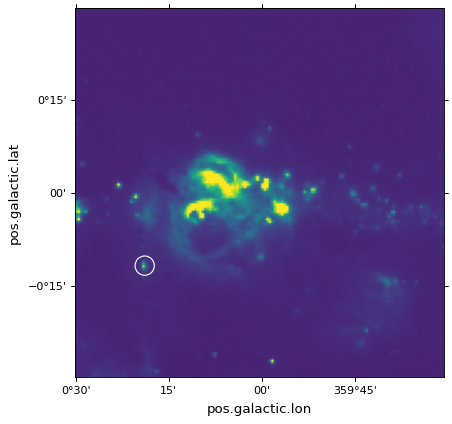In the case of `scatter()` and `plot()`, the positions of the center of the markers is transformed, but the markers themselves are drawn in the frame of reference of the image, which means that they will not look distorted.

## Patches/shapes/lines¶

Transformations can also be passed to Astropy or Matplotlib patches. For example, we can use the `get_transform()` method above to plot a quadrangle in FK5 equatorial coordinates:

```from astropy import units as u

r = Quadrangle((266.0, -28.9)*u.deg, 0.3*u.deg, 0.15*u.deg,
edgecolor='green', facecolor='none',
transform=ax.get_transform('fk5'))
```

(png, svg, pdf)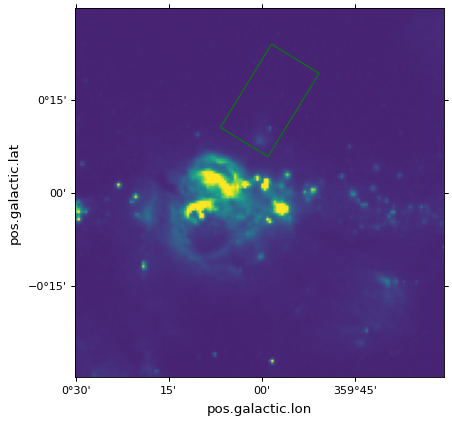In this case, the quadrangle will be plotted at FK5 J2000 coordinates (266deg, -28.9deg). See the Quadrangles section for more information on `Quadrangle`.

However, it is very important to note that while the height will indeed be 0.15 degrees, the width will not strictly represent 0.3 degrees on the sky, but an interval of 0.3 degrees in longitude (which, depending on the latitude, will represent a different angle on the sky). In other words, if the width and height are set to the same value, the resulting polygon will not be a square. The same applies to the `Circle` patch, which will not actually produce a circle:

```from matplotlib.patches import Circle

r = Quadrangle((266.4, -28.9)*u.deg, 0.3*u.deg, 0.3*u.deg,
edgecolor='cyan', facecolor='none',
transform=ax.get_transform('fk5'))

c = Circle((266.4, -29.1), 0.15, edgecolor='yellow', facecolor='none',
transform=ax.get_transform('fk5'))
```

(png, svg, pdf)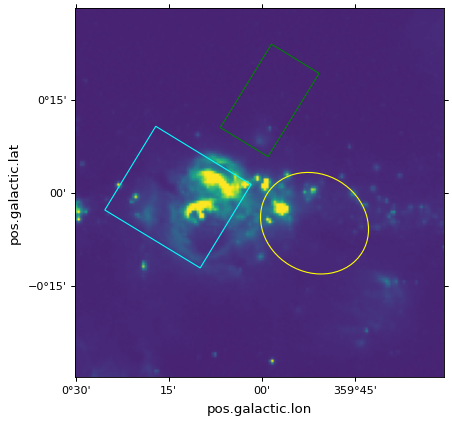Important

If what you are interested is simply plotting circles around sources to highlight them, then we recommend using `scatter()`, since for the circular marker (the default), the circles will be guaranteed to be circles in the plot, and only the position of the center is transformed.

To plot ‘true’ spherical circles, see the Spherical patches section.

`Quadrangle` is the recommended patch for plotting a quadrangle, as opposed to Matplotlib’s `Rectangle`. The edges of a quadrangle lie on two lines of constant longitude and two lines of constant latitude (or the equivalent component names in the coordinate frame of interest, such as right ascension and declination). The edges of `Quadrangle` will render as curved lines if appropriate for the WCS transformation. In contrast, `Rectangle` will always have straight edges. Here’s a comparison of the two types of patches for plotting a quadrangle in `ICRS` coordinates on `Galactic` axes:

```from matplotlib.patches import Rectangle

# Set the Galactic axes such that the plot includes the ICRS south pole
ax = plt.subplot(projection=wcs)
ax.set_xlim(0, 10000)
ax.set_ylim(-10000, 0)

# Overlay the ICRS coordinate grid
overlay = ax.get_coords_overlay('icrs')
overlay.grid(color='black', ls='dotted')

q = Quadrangle((255, -70)*u.deg, 100*u.deg, 20*u.deg,
transform=ax.get_transform('icrs'))

# Add a rectangle patch (100 degrees by 20 degrees)
r = Rectangle((255, -70), 100, 20,
label='Rectangle', edgecolor='red', facecolor='none', linestyle='--',
transform=ax.get_transform('icrs'))

plt.legend(loc='upper right')
```

(png, svg, pdf)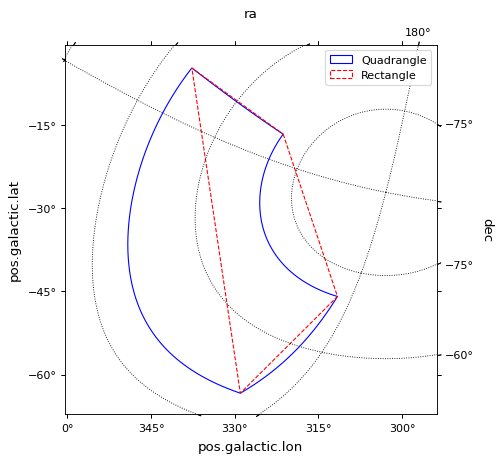## Contours¶

Overplotting contours is also simple using the `get_transform()` method. For contours, `get_transform()` should be given the WCS of the image to plot the contours for:

```filename = get_pkg_data_filename('galactic_center/gc_bolocam_gps.fits')
hdu = fits.open(filename)
levels=[1,2,3,4,5,6], colors='white')
```

(png, svg, pdf)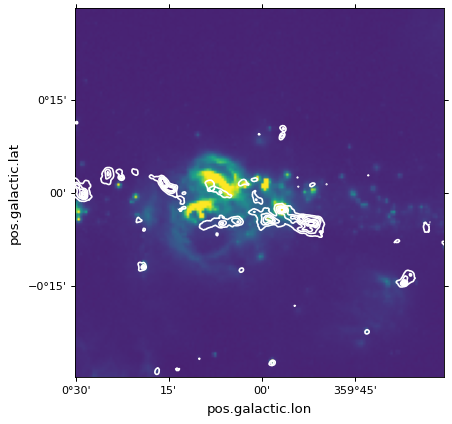## Spherical patches¶

In the case where you are making a plot of a celestial image, and want to plot a circle that represents the area within a certain angle of a longitude/latitude, the `Circle` patch is not appropriate, since it will result in a distorted shape (because longitude is not the same as the angle on the sky). For this use case, you can instead use `SphericalCircle`, which takes a tuple of `Quantity` or a `SkyCoord` object as the input, and a `Quantity` as the radius:

```from astropy import units as u
from astropy.coordinates import SkyCoord
from astropy.visualization.wcsaxes import SphericalCircle

r = SphericalCircle((266.4 * u.deg, -29.1 * u.deg), 0.15 * u.degree,
edgecolor='yellow', facecolor='none',
transform=ax.get_transform('fk5'))